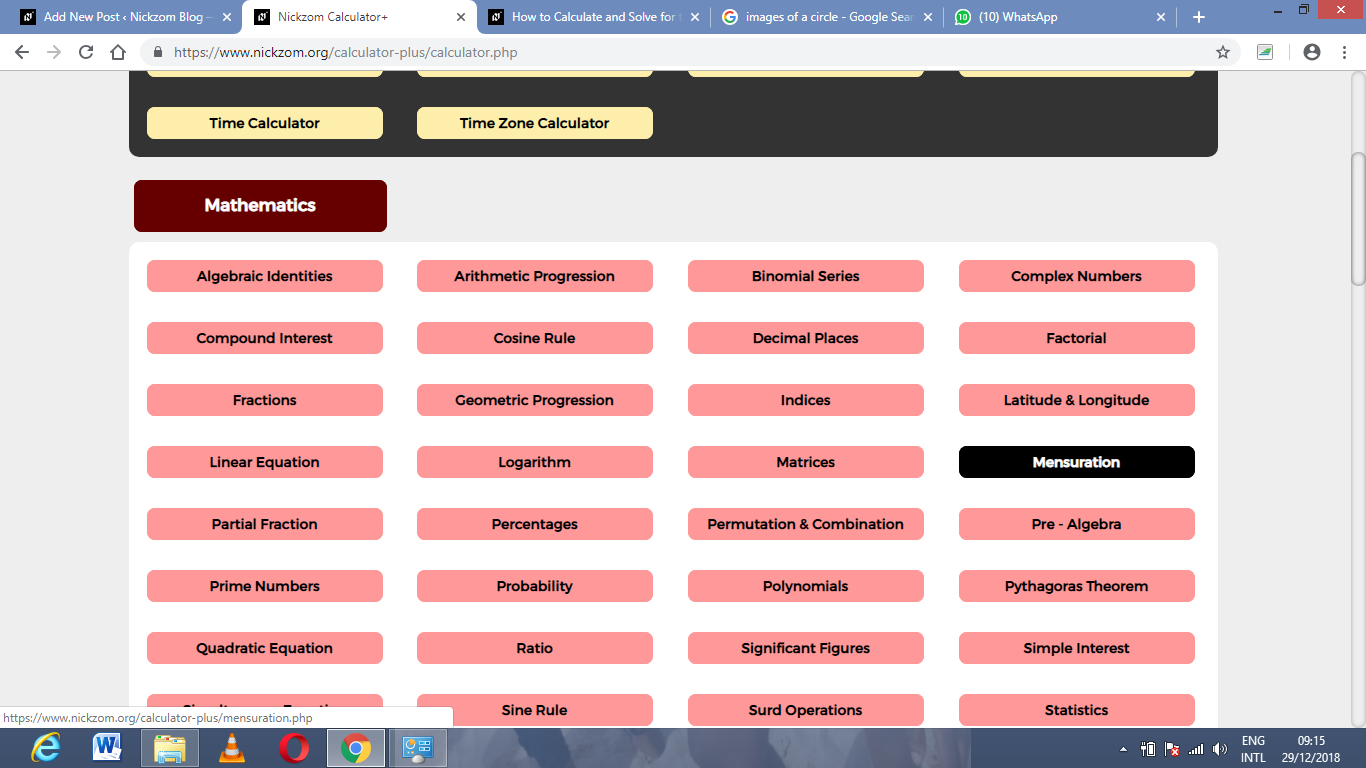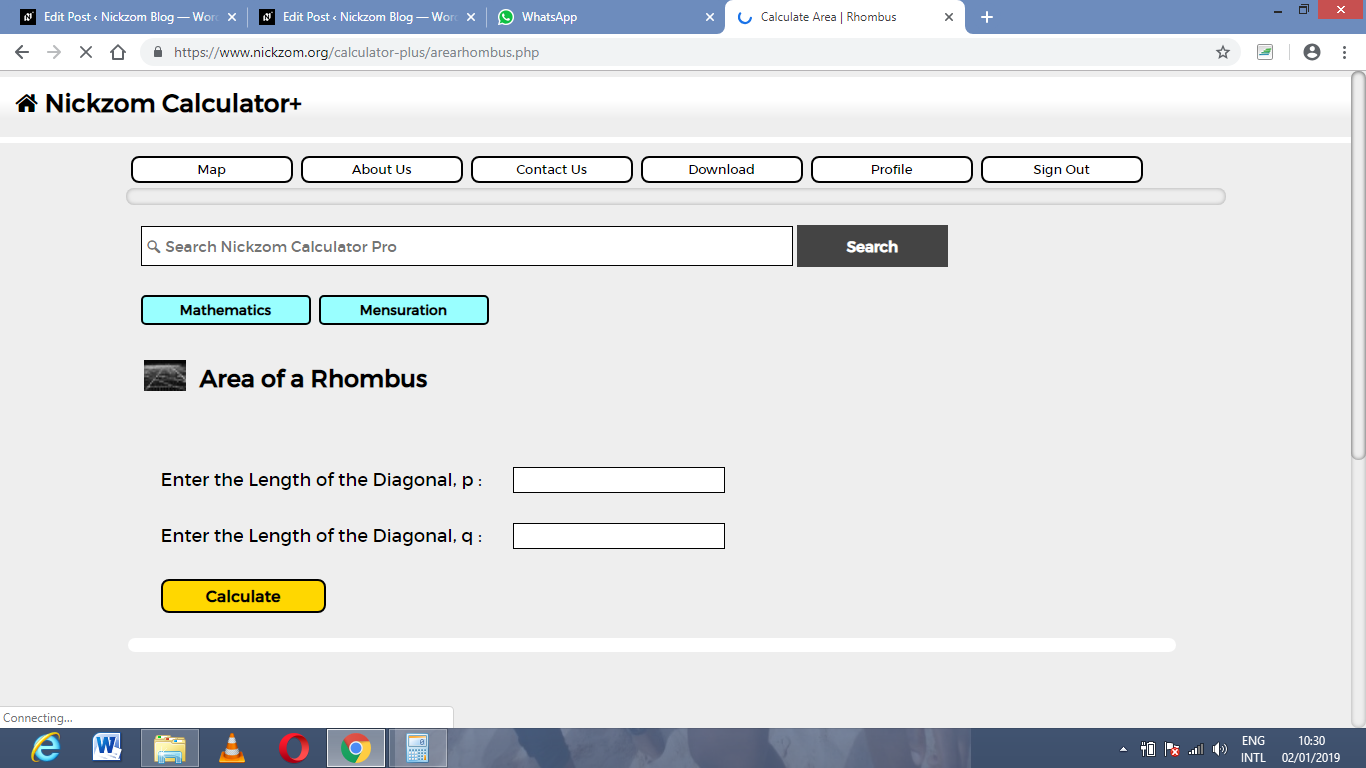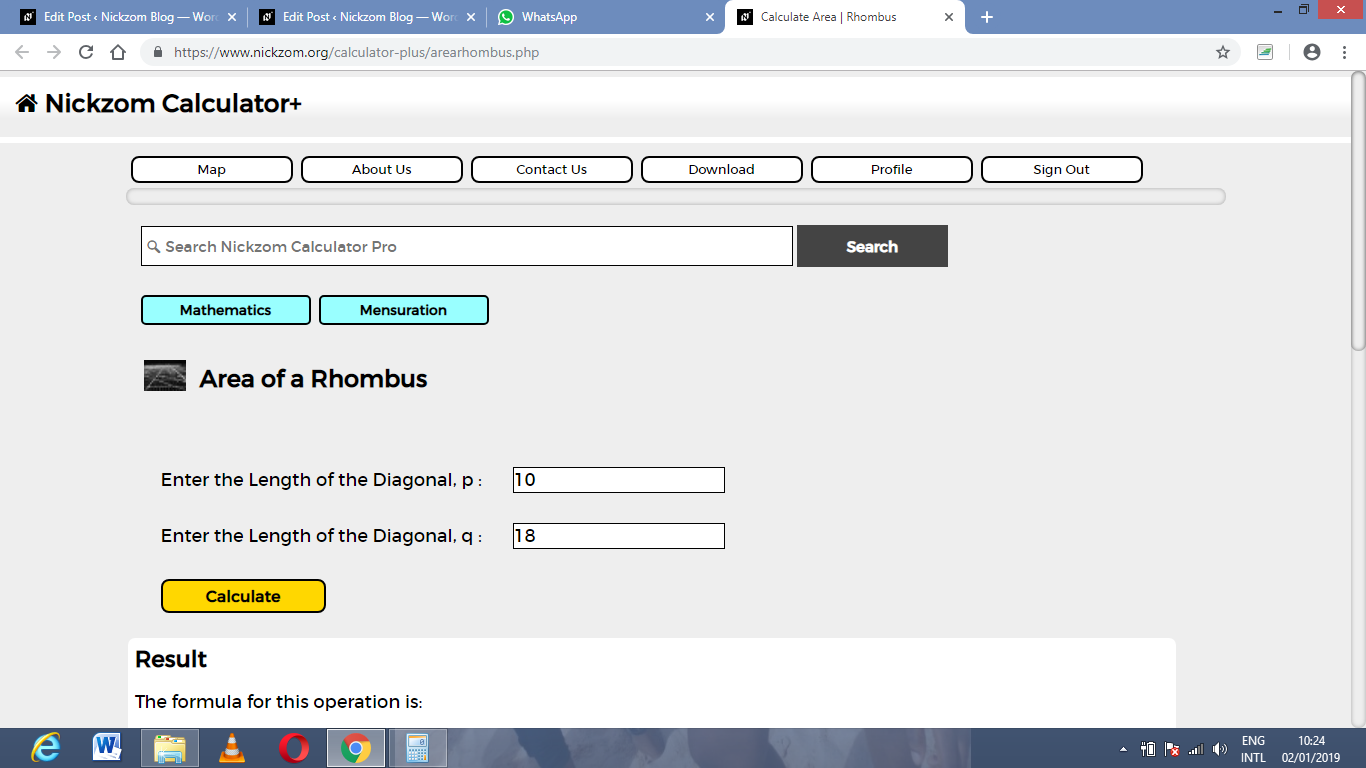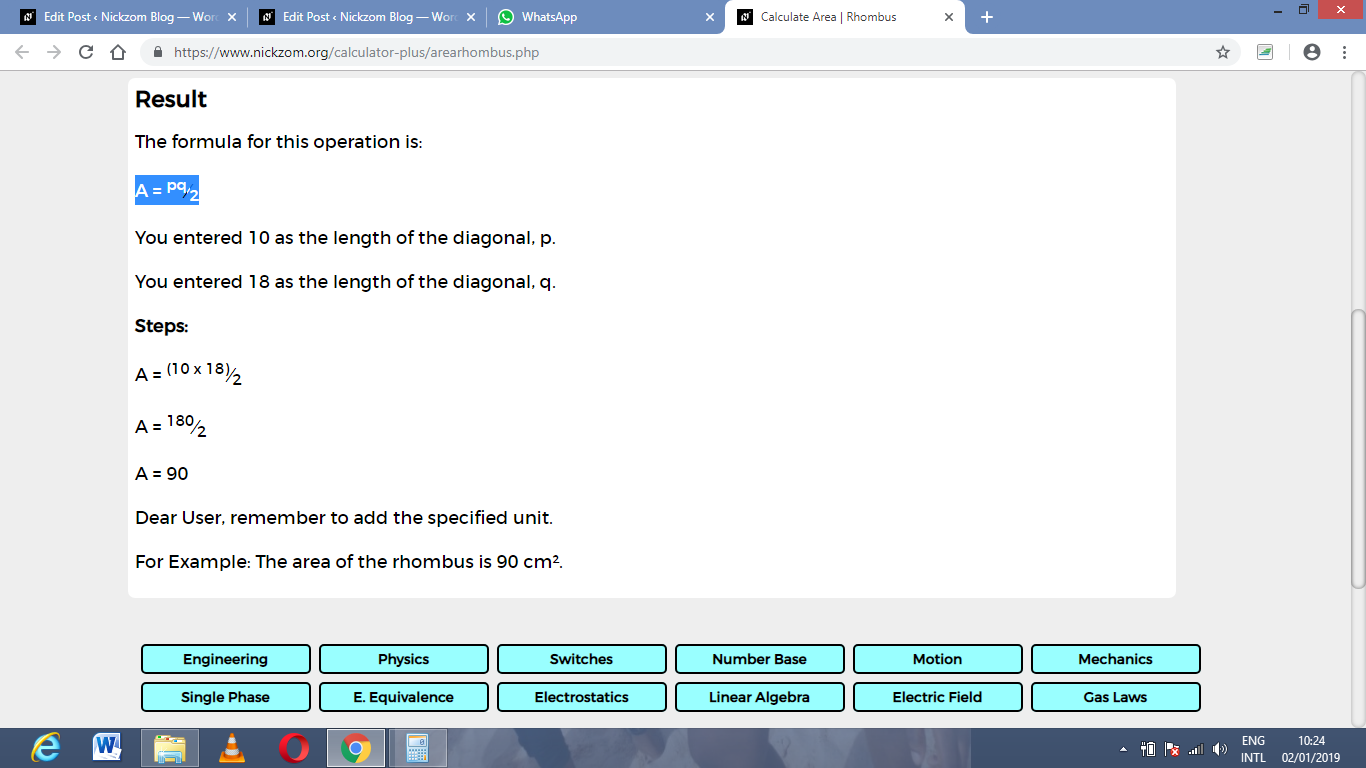# How to Calculate and Solve for the Length of the Diagonal and Area of a Rhombus | The Calculator Encyclopedia

The above image is a rhombus.

To compute the area of a rhombus, two essential parameters are needed and this parameters are length of the diagonal (p) and length of the diagonal (q).

The formula for calculating the area of a rhombus:

A = pq ⁄ 2

Where;
A = Area of the rhombus
p = length of the diagonal
q = length of the diagonal

Let’s solve an example;
Find the area of a rhombus when the length of the diagonal (p) is 10 cm and the length of the diagonal (q) is 18 cm.

This implies that;
p = length of the diagonal = 10 cm
q = length of the diagonal = 18 cm

A = pq2
A = 10 x 18 / 2
A = 180 / 2
A = 90

Therefore, the area of the rhombus is 90 cm2.

Calculating the length of the diagonal (p) using Area of the Rhombus and length of the diagonal (q).

p = 2A / q

Where;
A = Area of the rhombus
q = length of the diagonal

Let’s solve an example;
Given that the length of the diagonal (q) is 20 cm with an area of 60 cm2. Find the length of the diagonal (p)?

This implies that;
A = Area of the rhombus = 60 cm2
q = length of the diagonal = 20 cm

p = 2A / q
p = 2 x 60 / 20
p = 120 / 20
p = 6

Therefore, the length of the diagonal is 6 cm.

Calculating the length of the diagonal (q) using Area of rhombus and length of the diagonal (p).

q = 2A / p

Where;
A = Area of the rhombus
p = length of the diagonal

Let’s solve an example;
Given that the length of the diagonal (p) is 40 cm with an area of 90 cm2. Find the length of the diagonal (q)?

This implies that;
A = Area of the rhombus = 90 cm2
p = length of the diagonal = 40 cm

q = 2A / p
q = 2 x 90 / 40
q = 180 / 40
q = 4.5

Therefore the length of the diagonal (q) is 4.5 cm.

Nickzom Calculator – The Calculator Encyclopedia is capable of calculating the area of a rhombus.

To get the answer and workings of the area of a rhombus using the Nickzom Calculator – The Calculator Encyclopedia. First, you need to obtain the app.

You can get this app via any of these means:

To get access to the professional version via web, you need to register and subscribe for NGN 1,500 per annum to have utter access to all functionalities.
You can also try the demo version via https://www.nickzom.org/calculator

Once, you have obtained the calculator encyclopedia app, proceed to the Calculator Map, then click on Mensuration under the Mathematics sectionNow, click on Area of a Rhombus under MensurationThe screenshot below displays the page or activity to enter your values, to get the answer for the area of a rhombus according to the respective parameters which are the length of the diagonal (p) and length of the diagonal (q).Now, enter the values appropriately and accordingly for the parameters as required by the example above where the length of diagonal (p) is 10 cm and length of diagonal (q) is 18 cm.Finally, Click on CalculateAs you can see from the screenshot above, Nickzom Calculator – The Calculator Encyclopedia solves for the area of a rhombus and presents the formula, workings and steps too.